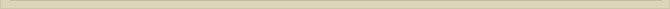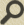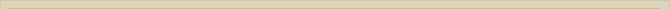## The Electromagnetic Mass

When you are interested in physics you must read “Unbelievable“!

In the chapter “The magnetic energy of a single moving charge” we demonstrated, with the help of the Electron Theory of Lorentz, that the magnetic energy (Wm) of a single moving bulb charge is equal to: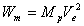, where Mp is the mass equivalence of the electrostatic energy of the charged bulb according to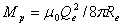.

In “The moving electron and magnetic energy” we calculated theoretically the magnetic energy of the “classical electron”. We derived the following equation for the magnetic energy of the moving electron: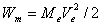Let us suggest that the electron, a charged mass, can be presented the energy/mass of a charged bulb and a, not yet identified (mechanical) part of the mass.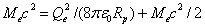The electrostatic mass of a charged bulb with the Compton radius explains exactly half the energy/mass of the electron. The magnetic energy of the moving charge equals the kinetic energy of the moving electron. The question to be answered is: “What kind of energy/mass presents the other half of the intrinsic energy of the electron?” We refer to the chapter “The Electron”, where the magnetic energy of the spin of the electron is calculated at exactly half the intrinsic energy of the electron.

The magnetic spin energy is responsible for and explains the physics why the charge of an electron is confined. The expanding force of the charged bulb of the electron is compensated means of the contracting force of the spinning magnetic field surrounding the electron. The charge of the electron is trapped.

The above presented E&M physics for the electron is however inconsistent with the QM perspectives and therefore must be addressed. We refer to the QM perspectives in “Lectures on Physics” part II chapter 28 “The Electromagnetic Mass” Feynman. In this chapter the electromagnetic mass of the electron is derived means of the momentum density (Equation 27.21 Lectures on Physics Volume II, Feynman).

g=εo ExB

According to the QM approach in (28-2) the magnitude of vector g is: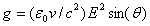because the momentum density vector is directed obliquely toward the line of motion (*).

Furthermore, and I quote (28-2): “The fields are symmetric about the line of motion, so when we integrate over space, the transverse components will sum to zero, giving a resultant momentum parallel to v. The component of g in this direction is g sin(θ), which we must integrate all over space.” (**)

In the above argumentation (*) and (**) vector p is thought to be partly compensated the opposite vector p. Vector summation is allowed in static situations, where the vectors for example express the magnitude and direction of a static force. p is however a dynamic vector, presenting the impulse of the moving mass/energy density at a certain point.

(For * and ** see Lectures on Physics Volume II, Feynman)

The total momentum p, according to the QM approach is then: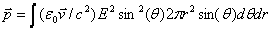where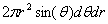is the volume element. The integration over all space gives: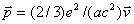(Equation 28.3 Feynman).

This equation, expressed in the symbols used in this article, gives the impulse: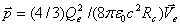The calculated electromagnetic mass according to equation 28.3 is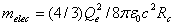, which is 4/3 the mass equivalence of the electric field or 2/3 the mass of the electron Me.

Although the QM approach differs from the approach in this article, the outcome should be consistent with each other. Because there is no consistency between the outcome of both approaches there must be an omission. In the QM approach the momentum density magnitude of vector g is diminished with the factor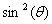, because of the previous mentioned and marked arguments (*) and (**).

To comprehend the effect of the correction of the magnitude of the momentum density g with the factorwe have to consider the QM equation for the total momentum; the integration of the momentum density g over space to p, in more detail (Feynman 28-2):The motion of the charge v, in the above equation, is independent of any variable in the equation, so we are allowed to abstract v out of the integral.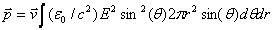Because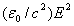presents the mass density (kg/m3) of the energy of the electrostatic field E and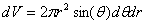is the volume element, the integration of the formula presents the calculation of the mass equivalent of the electrostatic field surrounding the charge. The corrections mentioned in (*) and (**) do not alter the implied physics of the integration. By integrating the momentum density g around the charge all over space, the physical interpretation of the integration is the calculation of the mass of the electrostatic field surrounding the charge. A mass is a scalar and therefore the correction of the magnitude of the momentum density vector with factorwill be an omission, which the conservation law for energy is violated. Part of the mass of the electrostatic field is unjustly ignored.

The magnitude of g presents the magnitude of the mass density impulse of the moving electrostatic field E in the direction of v. The direction of vector g does not present the direction of the movement of the electric field and therefore not the direction of the impulse. Compensation of the magnitude of g with factorviolates the energy conservation law because part of the mass/energy of the electrostatic field is then ignored.

The momentum density g at P as a result of the moving electrostatic field/energy Er with v at r should be:

g=Mr.v

where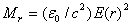presents the mass density (kg/m3) of the electrostatic field at r.

Integration of g over space gives:Equation (28.3 Feynman) is now: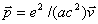This equation, expressed in the symbols used in this book, is: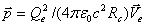or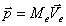or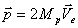(Me is the mass of the electron and Mp is the mass equivalence of the electrostatic field).

The corrected total momentum of the moving charge p, according to the QM approach, is now completely consistent with the derived equations in this chapter. The correctly derived electromagnetic mass, according to the QM approach, equals now the mass of the electron Me. Therefore the dynamic energy of the electron with the QM approach is also fully magnetic.

Electromagnetic Mass Lectures on Physics Volume II, Feynman

Next chapter: Discussion on magnetic and kinetic energy